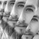5318 visitas
This study is an experiment utilizing the Ehlers Gaussian Filter technique combined with lag reduction techniques and true range to analyze trend activity.
Gaussian filters, as Ehlers explains it, are simply exponential moving averages applied multiple times.
First, beta and alpha are calculated based on the sampling period and number of poles specified. The maximum number of poles available in this script is 9.
Next, the data being analyzed is given a truncation option for reduced lag, which can be enabled with "Reduced Lag Mode".
Then the alpha and source values are used to calculate the filter and filtered true range of the dataset.
Filtered true range with a specified multiplier is then added to and subtracted from the filter, generating a channel.
Lastly, a one pole filter with a N pole alpha is averaged with the filter to generate a faster filter, which can be enabled with "Fast Response Mode".

Custom bar colors are included.

Note: Both the sampling period and number of poles directly affect how much lag the indicator has, and how smooth the output is.
Larger inputs will result in smoother outputs with increased lag, and smaller inputs will have noisier outputs with reduced lag.
For the best results, I recommend not setting the sampling period any lower than the number of poles + 1. Going lower truncates the equation.
Feb 16